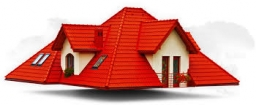# The roof

The house's roof has the shape of a regular quadrilateral pyramid 5 m high and the edge of the base 7 m. How many tiles with an area of 540 cm2 are needed?

n =  1583

### Step-by-step explanation:Did you find an error or inaccuracy? Feel free to write us. Thank you!

Tips to related online calculators
Do you want to convert area units?
Pythagorean theorem is the base for the right triangle calculator.

#### You need to know the following knowledge to solve this word math problem:

We encourage you to watch this tutorial video on this math problem: